# Roots of a polynomial

The roots of a polynomial are the values of $x$ that when substituted into the expression yield $0$. For example, the polynomial $x^2 - x$ has two roots, $0$ and $1$. A simple graph verifies this:

using CalculusWithJulia  # loads SymPy
using Plots
f(x) = x^2 - x
plot(f, -2, 2)
plot!(zero, -2, 2)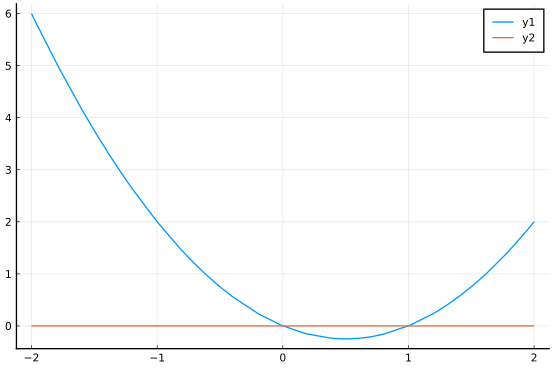The graph crosses the $x$-axis at both $0$ and $1$.

What is known about polynomial roots? Some simple questions might be:

• Will we always have a root?

• How many roots can there be?

• How large can the roots be?

We look at such questions here.

We begin with a comment that ties together two concepts related to polynomials. It allows us to speak of roots or factors interchangeably:

The factor theorem relates the roots of a polynomial with its factors: $r$ is a root of $p$ if and only if $(x-r)$ is a factor of the polynomial $p$.

Clearly, if $p$ is factored as $a(x-r_1) \cdot (x-r_2) \cdots (x - r_k)$ then each $r_i$ is a root, as a product involving at least one 0 term will be 0. The other implication is a consequence of polynomial division.

### Polynomial Division

Euclidean division division of integers $a, b$ uniquely writes $a = b\cdot q + r$ where $0 \leq r < |b|$. The quotient is $q$ and the remainder $r$. There is an analogy for polynomial division, where for two polynomial functions $f(x)$ and $g(x)$ it is possible to write

$~ f(x) = g(x) \cdot q(x) + r(x) ~$

where the degree of $r$ is less than the degree of $g(x)$. The long-division algorithm can be used to find both $q(x)$ and $r(x)$.

For the special case of a linear factor where $g(x) = x - c$, the remainder must be of degree $0$ (a constant) or the $0$ polynomial. The above simplifies to

$~ f(x) = (x-c) \cdot q(x) + r ~$

From this, we see that $f(c) = r$. Hence, when $c$ is a root of $f(x)$, then it must be that $r=0$ and so, $(x-c)$ is a factor.

The division algorithm for the case of linear term, $(x-c)$, can be carried out by the synthetic division algorithm. This algorithm produces $q(x)$ and $r$, a.k.a $f(c)$. The Wikipedia page describes the algorithm well.

The following is an example where $f(x) = x^4 + 2x^2 + 5$ and $g(x) = x-2$:

2 | 1 0 2  0  5
|   2 4 12 24
-------------
1 2 6 12 29

The polynomial $f(x)$ is coded in terms of its coefficients ($a_n$, $a_{n-1}$, $\dots$, $a_1$, $a_0$) and is written on the top row. The algorithm then proceeds from left to right. The number just produced on the bottom row is multiplied by $c$ and placed under the coefficient of $f(x)$. Then values are then added to produce the next number. The sequence produced above is 1 2 6 12 29. The last value (29) is $r=f(c)$, the others encode the coefficients of q(x), which for this problem is $q(x)=x^3 + 2x + 6 + 12$. That is, we have written:

$~ x^4 + 2x^2 + 5 = (x-2) \cdot (x^3 + 2x + 6 + 12) + 29. ~$

As $r$ is not $0$, we can say that $2$ is not a root of $f(x)$.

If we were to track down the computation that produced $f(2) = 29$, we would have

$~ 5 + 2 \cdot (0 + 2 \cdot (2 + 2 \cdot (0 + (2 \cdot 1)))) ~$

In terms of $c$ and the coefficients $x_0, x_1, x_2, x_3$, and $x_4$ this is

$~ x_0 + c\cdot(x_1 + c\cdot(x_2 + c\cdot(x_3 + c\cdot x_4))), ~$

The above pattern provides a means to compute $f(c)$ and could easily be generalized for higher degree polynomials. This generalization is called Horner's method. Horner's method has the advantage of also being faster and more accurate when floating point issues are accounted for. Julia has a built-in method to compute polynomial evaluations this way.

The SymPy package can carry out polynomial long division. The package was loaded with CalculusWithJulia. This command defines a variable that is assumed to be a real number:

@vars x real=true

(x,)


This nieve attempt to divide won't "just work" though:

(x^4 + 2x^2 + 5) / (x-2)

\begin{equation*}\frac{x^{4} + 2 x^{2} + 5}{x - 2}\end{equation*}

SymPy is fairly conservative in how it simplifies answers, and, as written, there is no compelling reason to change the expressions, though in our example we want it done.

For this task, divrem is available:

q, r = divrem(x^4 + 2x^2 + 5, x - 2)

(floor((x^4 + 2*x^2 + 5)/(x - 2)), x^4 + 2*x^2 - (x - 2)*floor((x^4 + 2*x^2
+ 5)/(x - 2)) + 5)


The answer is a tuple containing the quotient and remainder. The quotient itself could be found with div or ÷ and the remainder with rem.

As well, the apart function could be used for this task. This function computes the partial fraction decomposition of a ratio of polynomial functions.

apart((x^4 + 2x^2 + 5) / (x-2))

\begin{equation*}x^{3} + 2 x^{2} + 6 x + 12 + \frac{29}{x - 2}\end{equation*}

The function together would combine such terms, as an "inverse" to apart. This isn't so much of interest at the moment, but will be when techniques of integration are looked at.

### The rational root test

Factoring polynomials to find zeros is a task that most all readers here will recognize, and, perhaps, remember not so fondly. One helpful trick to find possible zeros by hand is the rational root theorem: if a polynomial has integer coefficients with $a_0 \neq 0$, than any rational root, $p/q$, must have $p$ dividing the constant $a_0$ and $q$ dividing the leading term $a_n$.

To glimpse why, suppose we have a polynomial with a rational root and integer coefficients. With this in mind, a polynomial with identical roots may be written as $(qx -p)(a_{n-1}x^{n-1}+\cdots a_1 x + a_0)$, where each coefficient is an integer. Multiplying through, we get that the polynomial is $qa_{n-1}x^n + \cdots + pa_0$. So $q$ is a factor of the leading coefficient and $p$ is a factor of the constant.

An immediate consequence is that if the polynomial with integer coefficients is monic, then any rational root must be an integer.

This gives a finite -though possibly large -set of values that can be checked to exhaust the possibility of a rational root. By hand this process can be tedious, though may be speeded up using synthetic division. This task is one of the mainstays of high school algebra where problems are chosen judiciously to avoid too many possibilities.

However, one of the great triumphs of computer algebra is the ability to factor polynomials with integer (or rational) coefficients over the rational numbers. This is typically done by first factoring over modular numbers (akin to those on a clock face) and has nothing to do with the rational root test.

SymPy can quickly find such a factorization, even for quite large polynomials with rational or integer coefficients.

For example, factoring $p = 2x^4 + x^3 -19x^2 -9x +9$. This has possible rational roots of plus or minus $1$ or $2$ divided by $1$, $3$, or $9$ - $12$ possible answers for this modest question. By hand that can be a bit of work, but factor does it without fuss:

p = 2x^4 + x^3 - 19x^2 - 9x + 9
factor(p)

\begin{equation*}\left(x - 3\right) \left(x + 1\right) \left(x + 3\right) \left(2 x - 1\right)\end{equation*}

### Fundamental theorem of algebra

There is a basic fact about the roots of a polynomial of degree $n$. Before formally stating it, we consider the earlier observation that a polynomial of degree $n$ for large values of $x$ has a graph that looks like the leading term. However, except at $0$, monomials do not cross the $x$ axis, the roots must be result of the interaction of lower order terms. Intuitively, since each term can contribute only one basic shape up or down, there can't be arbitrarily many roots. In fact, a consequence of the Fundamental Theorem of Algebra (Gauss) is:

A polynomial of degree $n$ has at most $n$ real roots.

This statement can be proved with the factor theorem and the division algorithm.

In fact the fundamental theorem states that there are exactly $n$ roots, though, in general, one must consider multiple roots and possible complex roots to get all $n$. (Consider $x^2$ to see why multiplicity must be accounted for and $x^2 + 1$ to see why complex values may be necessary.)

(The special case of the $0$ polynomial having no degree defined eliminates needing to exclude it, as it has infinitely many roots. Otherwise, the language would be qualified to have $n \geq 0$.)

## Finding roots of a polynomial

Knowing that a certain number of roots exist and actually finding those roots are different matters. For the simplest cases (the linear case) with $a_0+a_1x$, we know by solving algebraically that the root is $-a_0/a_1$. (We assume $a_1\neq 0$.) Of course, when $a_1 \neq 0$, the graph of the polynomial will be a line with some non-zero slope, so will cross the $x$-axis as the line and this axis are not parallel.

For the quadratic case, there is the famous quadratic formula (known since 2000 BC) to find the two roots guaranteed by the formula:

$~ \frac{-b \pm \sqrt{b^2 - 4ac}}{2a}. ~$

The discriminant is defined as $b^2 - 4ac$. When this is negative, the square root requires the concept of complex numbers to be defined, and the formula shows the two complex roots are conjugates. When the discriminant is $0$, then the root has multiplicity two, e.g., the polynomial will factor as $a_2(x-r)^2$. Finally, when the discriminant is positive, there will be two distinct, real roots. This figure shows the 3 cases, that are illustrated by $x^2 -1$, $x^2$ and $x^2 + 1$:

plot(x^2 - 1,  -2, 2, legend=false)  # two roots
plot!(x^2, -2, 2)                           # one (double) root
plot!(x^2 + 1, -2, 2)                       # no real root
plot!(zero, -2, 2)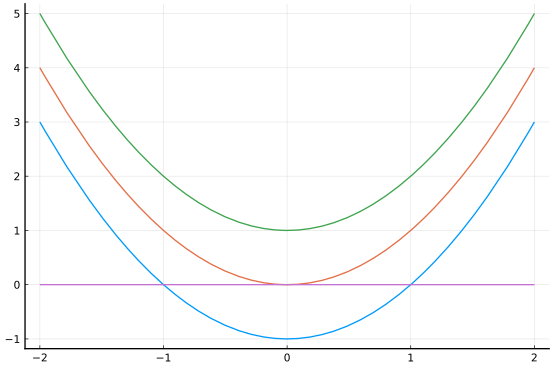There are similar formulas for the cubic and quartic cases. (The cubic formula was known to Cardano in 1545, though through Tartagli, and the quartic was solved by Ferrari, Cardano's roommate.)

In general, there is no such formula using radicals for 5th degree polynomials or higher, a proof first given by Ruffini in 1803 with improvement by Abel in 1824. Even though the fundamental theorem shows that any polynomial can be factored into linear and quadratic terms, there is no general method as to how. (It is the case that some such polynomials may be solvable by radicals, just not all of them.)

Finding roots with SymPy can also be done through its solve function, a function which also has a more general usage, as it can solve simple expressions or more than one expression. Here we illustrate that solve can easily handle quadratic expressions:

solve(x^2 + 2x - 3)

$\left[ \begin{array}{r}-3\\1\end{array} \right]$

The answer is a vector of values that when substituted in for the free variable x produce 0. The call to solve does not have an equals sign. To solve a more complicated expression of the type $f(x) = g(x)$, one can solve $f(x) - g(x) = 0$ or use the Eq function.

When the expression to solve has more than one free variable, the variable to solve for should be explicitly stated with a second argument. For example, here we show that solve is aware of the quadratic formula:

@vars a b c
solve(a*x^2 + b*x + c, x)

$\left[ \begin{array}{r}\frac{- b + \sqrt{- 4 a c + b^{2}}}{2 a}\\- \frac{b + \sqrt{- 4 a c + b^{2}}}{2 a}\end{array} \right]$

The solve function will respect assumptions made when a variable is defined through symbols or @vars:

@vars a
@vars b real=true
c = symbols("c", positive=true)
solve(a^2 + 1)     # works, as a can be complex

$\left[ \begin{array}{r}- i\\i\end{array} \right]$
solve(b^2 + 1)     # fails, as b is assumed real

Any[]

solve(c + 1)       # fails, as c is assumed positive

Any[]


Previously, it was mentioned that factor only factors polynomials with integer coefficients over rational roots. However, solve can be used to factor. Here is an example:

p = x^2 - 2
factor(p)

\begin{equation*}x^{2} - 2\end{equation*}

Nothing is found, as the roots are $\pm \sqrt{2}$, irrational numbers.

rts = solve(p)
prod(x-r for r in rts)

\begin{equation*}\left(x - \sqrt{2}\right) \left(x + \sqrt{2}\right)\end{equation*}

Solving cubics and quartics can be done exactly using radicals. For example, here we see the solutions to a quartic equation can be quite involved, yet still explicit. (We redefine x so that complex-valued solutions, if any, will be found.)

@vars x
solve(x^4 - 2x - 1)

$\left[ \begin{array}{r}- \frac{\sqrt{- \frac{2}{3 \sqrt{\frac{1}{4} + \frac{\sqrt{129}}{36}}} + 2 \sqrt{\frac{1}{4} + \frac{\sqrt{129}}{36}}}}{2} - \frac{\sqrt{- \frac{4}{\sqrt{- \frac{2}{3 \sqrt{\frac{1}{4} + \frac{\sqrt{129}}{36}}} + 2 \sqrt{\frac{1}{4} + \frac{\sqrt{129}}{36}}}} - 2 \sqrt{\frac{1}{4} + \frac{\sqrt{129}}{36}} + \frac{2}{3 \sqrt{\frac{1}{4} + \frac{\sqrt{129}}{36}}}}}{2}\\- \frac{\sqrt{- \frac{2}{3 \sqrt{\frac{1}{4} + \frac{\sqrt{129}}{36}}} + 2 \sqrt{\frac{1}{4} + \frac{\sqrt{129}}{36}}}}{2} + \frac{\sqrt{- \frac{4}{\sqrt{- \frac{2}{3 \sqrt{\frac{1}{4} + \frac{\sqrt{129}}{36}}} + 2 \sqrt{\frac{1}{4} + \frac{\sqrt{129}}{36}}}} - 2 \sqrt{\frac{1}{4} + \frac{\sqrt{129}}{36}} + \frac{2}{3 \sqrt{\frac{1}{4} + \frac{\sqrt{129}}{36}}}}}{2}\\\frac{\sqrt{- \frac{2}{3 \sqrt{\frac{1}{4} + \frac{\sqrt{129}}{36}}} + 2 \sqrt{\frac{1}{4} + \frac{\sqrt{129}}{36}}}}{2} + \frac{\sqrt{- 2 \sqrt{\frac{1}{4} + \frac{\sqrt{129}}{36}} + \frac{2}{3 \sqrt{\frac{1}{4} + \frac{\sqrt{129}}{36}}} + \frac{4}{\sqrt{- \frac{2}{3 \sqrt{\frac{1}{4} + \frac{\sqrt{129}}{36}}} + 2 \sqrt{\frac{1}{4} + \frac{\sqrt{129}}{36}}}}}}{2}\\- \frac{\sqrt{- 2 \sqrt{\frac{1}{4} + \frac{\sqrt{129}}{36}} + \frac{2}{3 \sqrt{\frac{1}{4} + \frac{\sqrt{129}}{36}}} + \frac{4}{\sqrt{- \frac{2}{3 \sqrt{\frac{1}{4} + \frac{\sqrt{129}}{36}}} + 2 \sqrt{\frac{1}{4} + \frac{\sqrt{129}}{36}}}}}}{2} + \frac{\sqrt{- \frac{2}{3 \sqrt{\frac{1}{4} + \frac{\sqrt{129}}{36}}} + 2 \sqrt{\frac{1}{4} + \frac{\sqrt{129}}{36}}}}{2}\end{array} \right]$

Some fifth degree polynomials are solvable in terms of radicals, however, solve will not seem to have luck with this particular fifth degree polynomial:

solve(x^5 - x + 1)

$\left[ \begin{array}{r}\operatorname{CRootOf} {\left(x^{5} - x + 1, 0\right)}\\\operatorname{CRootOf} {\left(x^{5} - x + 1, 1\right)}\\\operatorname{CRootOf} {\left(x^{5} - x + 1, 2\right)}\\\operatorname{CRootOf} {\left(x^{5} - x + 1, 3\right)}\\\operatorname{CRootOf} {\left(x^{5} - x + 1, 4\right)}\end{array} \right]$

(Though there is no formula involving only radicals like the quadratic equation, there is a formula for the roots in terms of a function called the Bring radical.)

### Numerically finding roots

The solve function can be used to get numeric approximations to the roots. It is as easy as calling N on the solutions:

rts = solve(x^5 - x + 1)
N.(rts)     # note the .( to broadcast over all values in rts

5-element Array{Number,1}:
-1.167303978261418684256045899854842180720560371525489
039140082449275651903429536
-0.18123244446987538 - 1.0839541013177107im
-0.18123244446987538 + 1.0839541013177107im
0.7648844336005847 - 0.35247154603172626im
0.7648844336005847 + 0.35247154603172626im


Here we see another example:

ex = x^7 -3x^6 +  2x^5 -1x^3 +  2x^2 + 1x^1  - 2
solve(ex)

$\left[ \begin{array}{r}1\\2\\\operatorname{CRootOf} {\left(x^{5} - x - 1, 0\right)}\\\operatorname{CRootOf} {\left(x^{5} - x - 1, 1\right)}\\\operatorname{CRootOf} {\left(x^{5} - x - 1, 2\right)}\\\operatorname{CRootOf} {\left(x^{5} - x - 1, 3\right)}\\\operatorname{CRootOf} {\left(x^{5} - x - 1, 4\right)}\end{array} \right]$

This finds two of the seven roots, the remainder can be found numerically:

N.(solve(ex))

7-element Array{Number,1}:
1
2
1.1673039782614186842560458998548421807205603715254890
39140082449275651903429536
-0.7648844336005847 - 0.35247154603172626im
-0.7648844336005847 + 0.35247154603172626im
0.18123244446987538 - 1.0839541013177107im
0.18123244446987538 + 1.0839541013177107im


### The solveset function

SymPy is phasing in the solveset function to replace solve. The main reason being that solve has too many different output types. The output of solveset is always a set. For tasks like this, we use the elements function to access the individual answers. To illustrate:

p = 8x^4 - 8x^2  + 1
rts = solveset(p)

\begin{equation*}\left\{- \sqrt{\frac{1}{2} - \frac{\sqrt{2}}{4}}, \sqrt{\frac{1}{2} - \frac{\sqrt{2}}{4}}, - \sqrt{\frac{\sqrt{2}}{4} + \frac{1}{2}}, \sqrt{\frac{\sqrt{2}}{4} + \frac{1}{2}}\right\}\end{equation*}

The rts object does not allow immediate access to its elements. For that, as rts is a finite set, elements will work to return a vector:

elements(rts)

$\left[ \begin{array}{r}- \sqrt{\frac{\sqrt{2}}{4} + \frac{1}{2}}\\- \sqrt{\frac{1}{2} - \frac{\sqrt{2}}{4}}\\\sqrt{\frac{\sqrt{2}}{4} + \frac{1}{2}}\\\sqrt{\frac{1}{2} - \frac{\sqrt{2}}{4}}\end{array} \right]$

So to get the numeric approximation is

N.(elements(solveset(p)))

4-element Array{BigFloat,1}:
-0.92387953251128675612818318939678828682241662586364248611509773128053500
75011054
-0.38268343236508977172845998403039886676134456248562704143380063562754603
39600903
0.92387953251128675612818318939678828682241662586364248611509773128053500
75011054
0.38268343236508977172845998403039886676134456248562704143380063562754603
39600903


## Do numeric methods matter when you can just graph?

It may seem that certain practices related to roots of polynomials are unnecessary as we could just graph the equation and look for the roots. This feeling is perhaps motivated by the examples given in textbooks to be worked by hand, which necessarily focus on smallish solutions. But, in general, without some sense of where the roots are, an informative graph itself can be hard to produce. That is, technology doesn't displace thinking - it only supplements it.

Take for example, the polynomial $(x-20)^5 - (x-20) + 1$. In this form we might think the roots are near 20. However, were we presented with this polynomial in expanded form: $x^5 - 100x^4 + 4000x^3 - 80000x^2 + 799999x - 3199979$, we might be tempted to just graph it to find roots. A naive graph might be to plot over $[-10, 10]$:

p =   x^5 - 100x^4 + 4000x^3 - 80000x^2 + 799999x - 3199979
plot(p, -10, 10)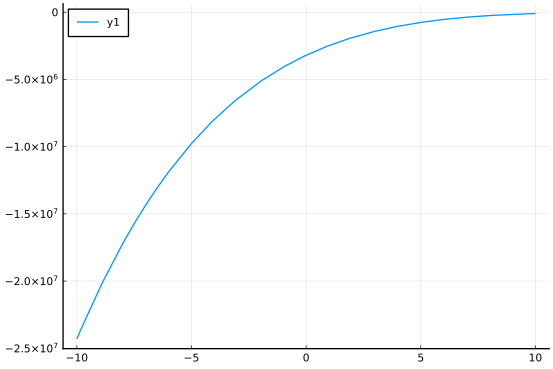This seems to indicate a root near 10. But look at the scale of the $y$ axis. The value at $-10$ is around $-25,000,000$ so it is really hard to tell if $f$ is near $0$ when $x=10$, as the range is too large.

A graph over $[10,20]$ is still unclear:

plot(p, 10,20)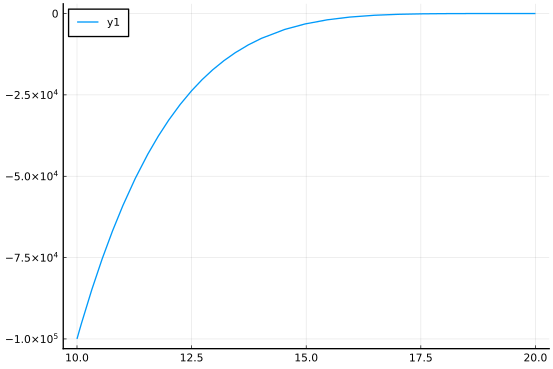We see that what looked like a zero near 10, was actually a number around $-100,000$.

Continuing, a plot over $[15, 20]$ still isn't that useful. It isn't until we get close to 18 that the large values of the polynomial allow a clear vision of the values near $0$. That being said, plotting anything bigger than 22 quickly makes the large values hide those near $0$, and might make us think where the function dips back down there is a second or third zero, when only 1 is the case. (We know that, as this is the same $x^5 - x + 1$ shifted to the right by 20 units.)

plot(p, 18, 22)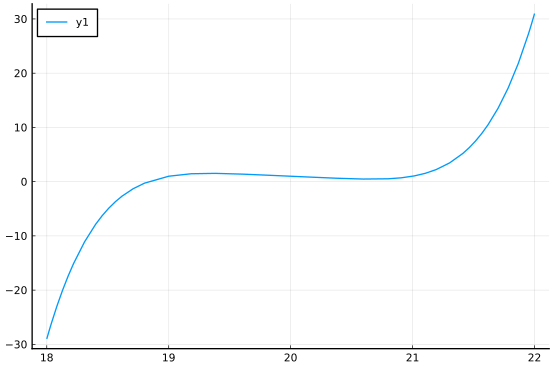Not that it can't be done, but graphically solving for a root here can require some judicious choice of viewing window. Even worse is the case where something might graphically look like a root, but in fact not be a root. Something like $(x-100)^2 + 0.1$ will demonstrate.

The point of this is to say, that it is useful to know where to look for roots, even if graphing calculators or graphing programs make drawing graphs relatively painless. A better way in this case would be to find the real roots first, and then incorporate that information into the choice of plot window.

## Some facts about the real roots of a polynomial

A polynomial with real coefficients may or may not have real roots. The following discusses some simple checks on the number of real roots and bounds on how big they can be. This can be roughly used to narrow viewing windows when graphing polynomials.

### Descartes' rule of signs

The study of polynomial roots is an old one. In 1637 Descartes published a simple method to determine an upper bound on the number of positive real roots of a polynomial.

Descartes' rule of signs. if $p=a_n x^n + a_{n-1}x^{n-1} + \cdots a_1x + a_0$ then the number of positive real roots is either equal to the number of sign differences between consecutive nonzero coefficients, or is less than it by an even number. Repeated roots are counted separately.

One method of proof first shows that in synthetic division by $(x-c)$ with $c > 0$, we must have that any sign change in $q$ is related to a sign change in $p$ and there must be at least one less. This is then used to show that there can be only as many positive roots as sign changes. That the difference comes in pairs is related to complex roots of real polynomials always coming in pairs. A formal proof is given here.

An immediate consequence, is that a polynomial whose coefficients are all non-negative will have no positive real roots.

Applying this to the polynomial $x^5 -x + 1$ we get that the sign pattern is +, -, + which has two changes of sign. The number of positive real roots is either 2 or 0. In fact there are $0$ for this case.

What about negative roots? Cleary, any negative root of $p$ is a positive root of $q(x) = p(-x)$, as the graph of $q$ is just that of $p$ flipped through the $y$ axis. But the coefficients of $q$ are the same as $p$, except for the odd-indexed coefficients ($a_1, a_3, \dots$) have a changed sign. Continuing with our example, for $q(x) = -x^5 + x + 1$ we get the new sign pattern -, +, + which yields one sign change. That is, there must be a negative real root, and indeed there is, $x \approx -1.1673$.

For another example, if we looked at $f(x) = x^5 - 100x^4 + 4000x^3 - 80000x^2 + 799999x - 3199979$ again, we see that there could be 1, 3, or 5 positive roots. However, changing the signs of the odd powers leaves all "-" signs, so there are $0$ negative roots. From the graph, we saw just 1 real root, not 3 or 5. We can verify numerically with:

p =  x^5 - 100x^4 + 4000x^3 - 80000x^2 + 799999x - 3199979
N.(solve(p))

5-element Array{Number,1}:
18.83269602173858131574395410014515781927943962847451096
085991755072434809657041
19.818767555530126 - 1.0839541013177107im
19.818767555530126 + 1.0839541013177107im
20.764884433600585 - 0.35247154603172626im
20.764884433600585 + 0.35247154603172626im


### Cauchy's bound on the magnitude of the real roots.

Descartes' rule gives a bound on how many real roots there may be. Cauchy provided a bound on how large they can be. Assume our polynomial is monic (if not, divide by $a_n$ to make it so, as this won't effect the roots). Then any real root is no larger in absolute value than $|a_0| + |a_1| + |a_2| + \cdots + |a_n|$, (this is expressed in different ways.)

To see precisely why this bound works, suppose $x$ is a root with $|x| > 1$ and let $h$ be the bound. Then since $x$ is a root, we can solve for $x^n$ as:

$~ x_n = -(a_0 + a_1 x + \cdots a_{n-1}x^{n-1}) ~$

Which after taking absolute values of both sides, yields:

$~ |x_n| \leq |a_0| + |a_1||x| + |a_2||x^2| + \cdots |a_{n-1}| |x^{n-1}| \leq (h-1) (1 + |x| + |x^2| + \cdots |x^{n-1}|). ~$

The last sum can be computed using a formula for geometric sums, $(|x^n| - 1)/(|x|-1)$. Rearranging, gives the inequality:

$~ |x| - 1 \leq (h-1) \cdot (1 - \frac{1}{|x^n|} ) \leq (h-1) ~$

from which it follows that $|x| \leq h$, as desired.

For our polynomial $x^5 -x + 1$ we have the sum above is $3$. The lone real root is approximately $-1.1673$ which satisfies $|-1.1673| \leq 3$.

## Questions

###### Question

What is the remainder of dividing $x^4 - x^3 - x^2 + 2$ by $x-2$?

###### Question

What is the remainder of dividing $x^4 - x^3 - x^2 + 2$ by $x^3 - 2x$?

###### Question

We have that $x^5 - x + 1 = (x^3 + x^2 - 1) \cdot (x^2 - x + 1) + (-2x + 2)$.

What is the remainder of dividing $x^5 - x + 1$ by $x^2 - x + 1$?

###### Question

Consider this output from synthetic division

2 | 1 0 0 0 -1  1
|   2 4 8 16 30
---------------
1 2 4 8 15 31

representing $p(x) = q(x)\cdot(x-c) + r$.

What is $p(x)$?

What is $q(x)$?

What is $r$?

###### Question

Let $p=x^4 -9x^3 +30x^2 -44x + 24$

Factor $p$. What are the factors?

###### Question

Does the expression $x^4 - 5$ factor over the rational numbers?

Using solve, how many real roots does $x^4 - 5$ have:

###### Question

What are the numeric values of the real roots of $f(x) = x^6 - 5x^5 + x^4 - 3x^3 + x^2 - x + 1$?

###### Question

Odd polynomials must have at least one real root.

Consider the polynomial $x^5 - 3x + 1$. Does it have more than one real root?

Consider the polynomial $x^5 - 1.5x + 1$. Does it have more than one real root?

using Roots
xs = fzeros(x -> x^5 - 1.5x + 1, -10, 10)
yesnoq(length(xs) > 1)

###### Question

What is the maximum number of positive, real roots that Descarte's bound says $p=x^5 + x^4 - x^3 + x^2 + x + 1$ can have?

How many positive, real roots does it actually have?

What is the maximum number of negative, real roots that Descarte's bound says $p=x^5 + x^4 - x^3 + x^2 + x + 1$ can have?

How many negative, real roots does it actually have?

###### Question

Let $f(x) = x^5 - 4x^4 + x^3 - 2x^2 + x$. What does Cauchy's bound say is the largest possible magnitude of a root?

What is the largest magnitude of a real root?

###### Question

As $1 + 2 + 3 + 4$ is $10$, Cauchy's bound says that the magnitude of the largest real root of $x^3 - ax^2 + bx - c$ is $10$ where $a,b,c$ is one of $2,3,4$. By considering all 6 such possible polynomials (such as $x^3 - 3x^2 + 2x - 4$) what is the largest magnitude or a root?

###### Question

The roots of the Chebyshev polynomials are helpful for some numeric algorithms. These are a family of polynomials related by $T_{n+1}(x) = 2xT_n(x) - T_{n-1}(x)$ (a recurrence relation in the manner of the Fibonacci sequence). The first two are $T_0(x) = 1$ and $T_1(x) =x$.

• Based on the relation, figure out $T_2(x)$. It is

• True or false, the $degree$ of $T_n(x)$ is $n$: (Look at the defining relation and reason this out).

• The fifth one is $T_5(x) = 32x^5 - 32x^3 + 6x$. Cauchy's bound says that the largest root has absolute value

1 + 1 + 6/32

2.1875


The Chebyshev polynomials have the property that in fact all $n$ roots are real, distinct, and in $[-1, 1]$. Using SymPy, find the magnitude of the largest root:

• Plotting p over the interval $[-2,2]$ does not help graphically identify the roots:

plot(p, -2, 2)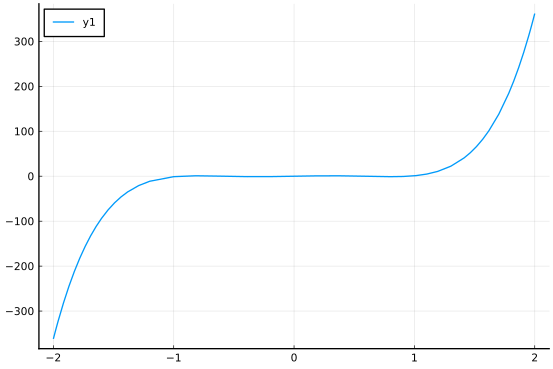Does graphing over $[-1,1]$ show clearly the $5$ roots?